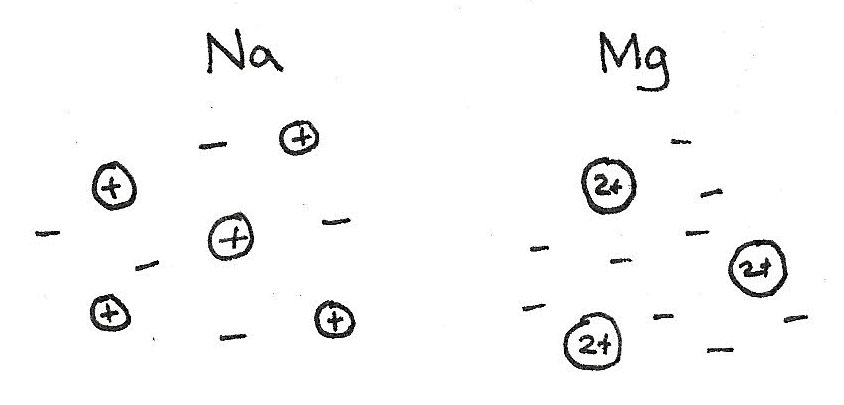# Periodic Table

In the periodic table, elements are arranged in order of atomic number.

• Each horizontal row is called a period. Trends are sometimes shown across a period, which is called periodicity.
• Each vertical column is called a group. These elements share similar chemical properties.

## Periodicity

This is the regular periodic variation of properties of elements with atomic number and position in the periodic table. These trends allow predictions to be made about the likely properties of an element.

Chemical reactions involve electrons in the outer shell. This is why elements in the same group have similar chemical properties as they all have the same number of electrons in the outer shell, with the same type of orbital.

## Ionisation Energies

Trends can be viewed on a periodic table:

#### Across a period

Across a period, the number of protons increase, increasing the attraction between the nucleus and the outer electrons. This decreases the atomic radius, and makes the first ionisation energy increase as more energy is needed to remove an outer shell electron. Electron shielding is unchanged however, as the outer electrons occupy the same outer shell.

#### Down a group

Down a group, the first ionisation energy decreases. Firstly, as the number of shells increases, the attractive force is weakened. Also with more shells, the electron shielding increases resulting in a weaker attraction between the nucleus and the outer shell electrons. While there are more protons with a stronger attractive force, these effects are outweighed by the increase in atomic radius and shielding.

## Melting and boiling points

Across period 3 the elements go from metals to non-metals. Between these two is $Si$ which is harder to classify as it has a shiny appearance like a metal although it is brittle. It also slightly conducts electricity. It is therefore classified as a metalloid.

The trend of melting and boiling points is caused by the types of bonding which are shown below.

### Metallic Bonding

At the left of the group there are $Na$, $Mg$ and $Al$. These have a giant metallic structure with strong electrostatic attractions between the ions and sea of delocalised electrons. Due to the increasing charge of these ions, the ionisation energies increase across these three elements.### Giant Covalent

$Si$ is the only element to have a giant covalent structure. This is where atoms are held together by strong covalent bonds in a lattice.

### Simple Molecular

$P_{4}$, $S_{8}$, $Cl_{2}$ and $Ar$ all exist as simple molecular structures with weak van der Waals' forces between the molecules.

This pattern across the period is also shown in period 2 with $Li$ and $Be$ metallically bonded, $B$ and $C$ covalently bonded in a lattice and $N_{2}$, $O_{2}$, $F_{2}$ and $Ne$ as simple molecular structures.